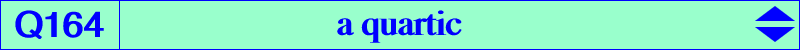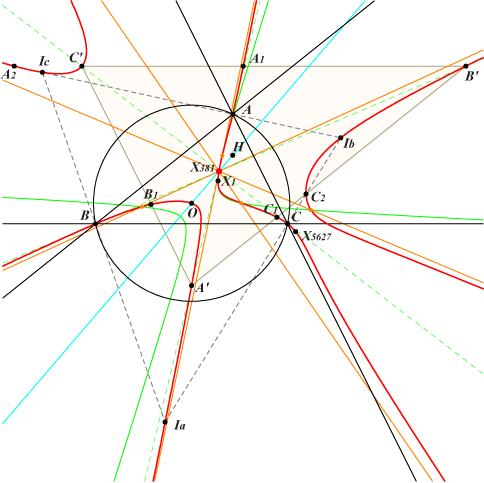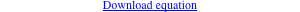too complicated to be written here. Click on the link to download a text file.X(1), X(3), X(381), X(5627) excenters other points belowLet P be a point with circumcevian triangle PaPbPc. Denote by Ea, Eb, Ec the centers X(381) of triangles PaBC, PbCA, PcAB respectively. Recall that X(381) is the midpoint of GH. PaPbPc and EaEbEc are perspective if and only if P lies on the quartic Q164 (together with the circumcircle). Q164 has four asymptotes concurring at X(381) and meeting the quartic again at four points lying on a conic passing through X(381) and the images of A, B, C under the homothety h(X381, 2/3). Other points on Q164 : • A', B', C' : images of A, B, C under the homothety h(X381, -2). This triangle A'B'C' is congruent to the antimedial triangle. • A1, B1, C1 and A2, B2, C2 : intersections of the sidelines of A'B'C' with the internal and external bisectors of ABC respectively. • two other points E1, E2 on the Euler line and on the image (C) of the polar circle under the homothety h(X4, √2). These points are obviously real when ABC is obtusangle. (C) is orthogonal to the circumcircle (O) hence E1, E2 are inverse in (O). See the related quartics Q162, Q163 and Q165. See Q023 for a generalization.